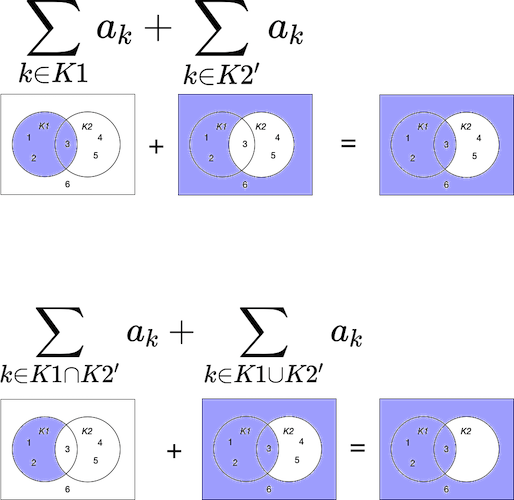# Concrete Mathematics: Summation Equation 2.20 visualised

In Concrete Mathematics (Graham, Knuth, Patashnik) we have the following preamble and equation 2.20

For example, here is an important rule for combining different sets of indices: If $K$ and $K’$ are any sets of integers, then:

$\sum_{k\in K}a_k + \sum_{k \in K'}a_k = \sum_{k \in K \cap K'}a_k + \sum_{k \in K \cup K'}a_k$

That this would be so was not inuitively obvious to me so I have to visualise it to really get a sense for it. We can see that the above equations are equal by using Venn diagrams. I have illustrated this below; the only difference is I used sets $K1$ and $K2$ instead of just $K$ representing any sets(Higher resolution image is available)

The RHS is equal in both cases.

Categories:

Updated: